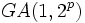No proper nontrivial transitively normal subgroup not implies simple

This article gives the statement and possibly, proof, of a non-implication relation between two group properties. That is, it states that every group satisfying the first group property (i.e., group having no proper nontrivial transitively normal subgroup) need not satisfy the second group property (i.e., simple group)
View a complete list of group property non-implications | View a complete list of group property implications
Get more facts about group having no proper nontrivial transitively normal subgroup|Get more facts about simple group

Statement

We can have a group that is not simple but has no proper nontrivial transitively normal subgroup.

Definitions used

Transitively normal subgroup

Further information: Transitively normal subgroup

A subgroup$H$ of a group$G$ is termed transitively normal in$G$ if whenever$K$ is a normal subgroup of$H$,$K$ is normal in$G$.

Simple group

Further information: Simple group

A$G$ is termed simple if$G$ has no proper nontrivial normal subgroup.

Proof

Example of the alternating group

Further information: alternating group:A4

Let$G$ be the alternating group on the set$\{ 1,2,3,4 \}$.$G$ has three normal subgroups: the whole group, the trivial subgroup, and the four-element subgroup:$H := \{ (), (1,2)(3,4), (1,3)(2,4), (1,4)(2,3) \}$.$H$ is not transitively normal, because the two-element subgroup:$K := \{ (), (1,2)(3,4) \}$

is normal in$H$ and not normal in$G$. Thus:

•$G$ is not simple since it has a proper nontrivial normal subgroup, namely$H$.
•$G$ has no proper nontrivial normal subgroup.

A more generic example

Let$p$ be such that$2^p - 1$ is a prime (in this case, it is a Mersenne prime). Consider the general affine group$GA(1,2^p)$: this is the semidirect product of the additive group of the field of order$2^p$ with its multiplicative group. The only proper nontrivial normal subgroup of this group is the additive group.

This subgroup is not transitively normal, because all its proper nontrivial subgroups are normal in it, but none of them are normal in the whole group. That is because the multiplicative group acts transitively on the non-identity elements, and hence cannot preserve any proper nontrivial subgroup.

The alternating group is a special case of the above where$p = 2$.